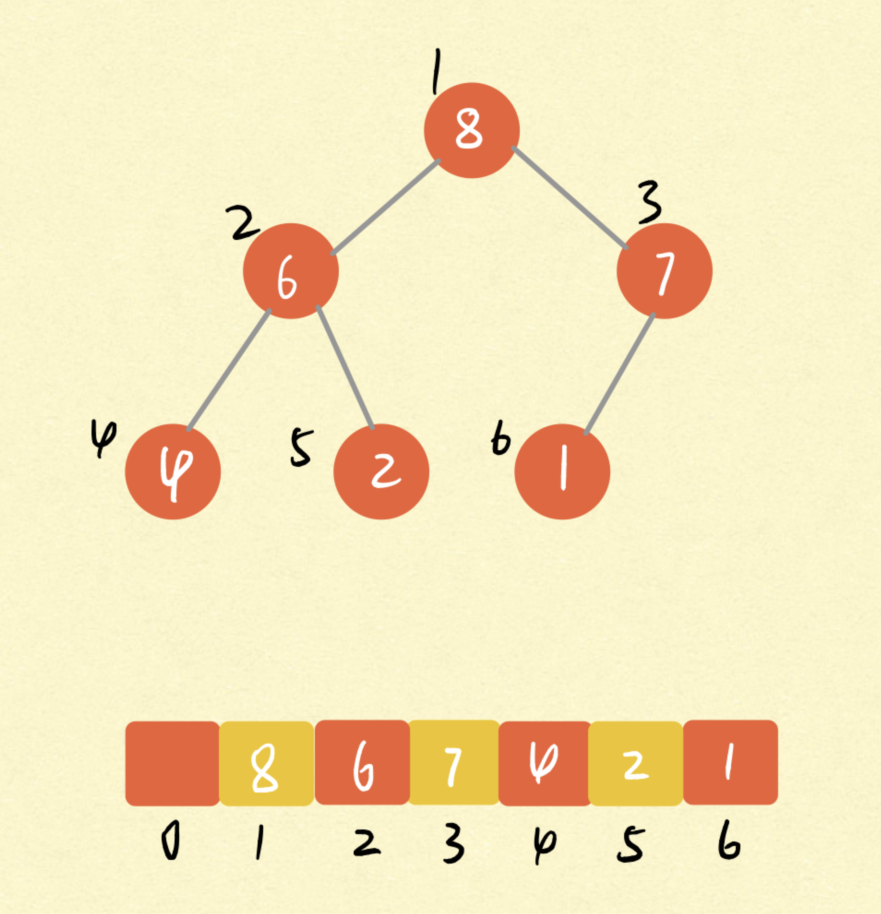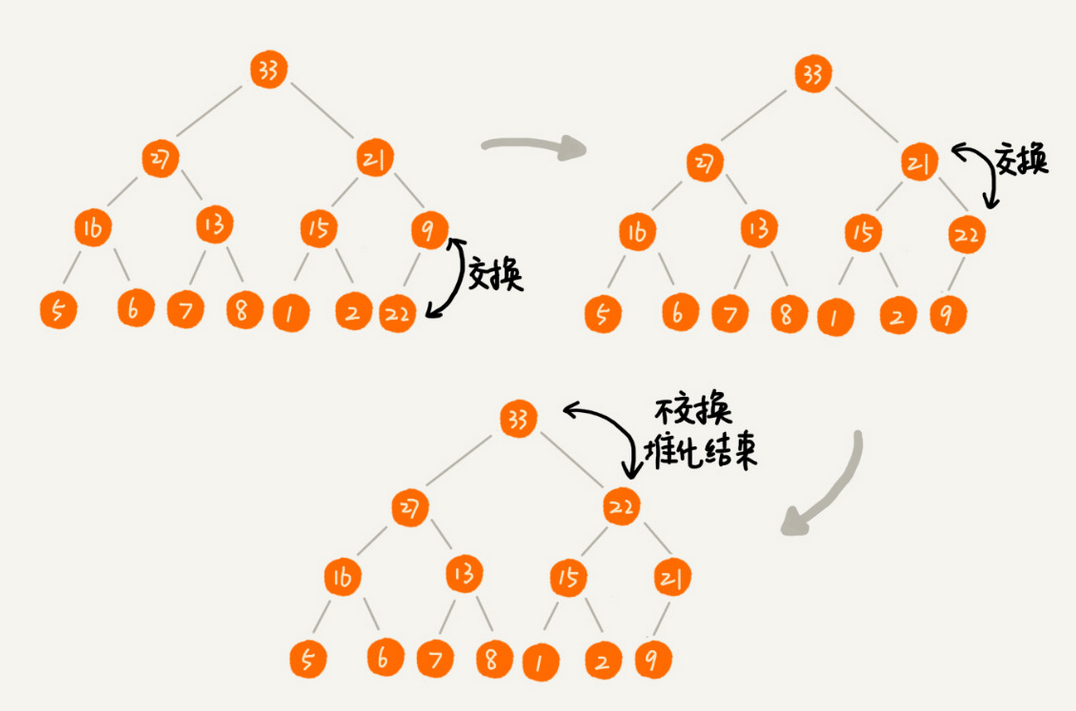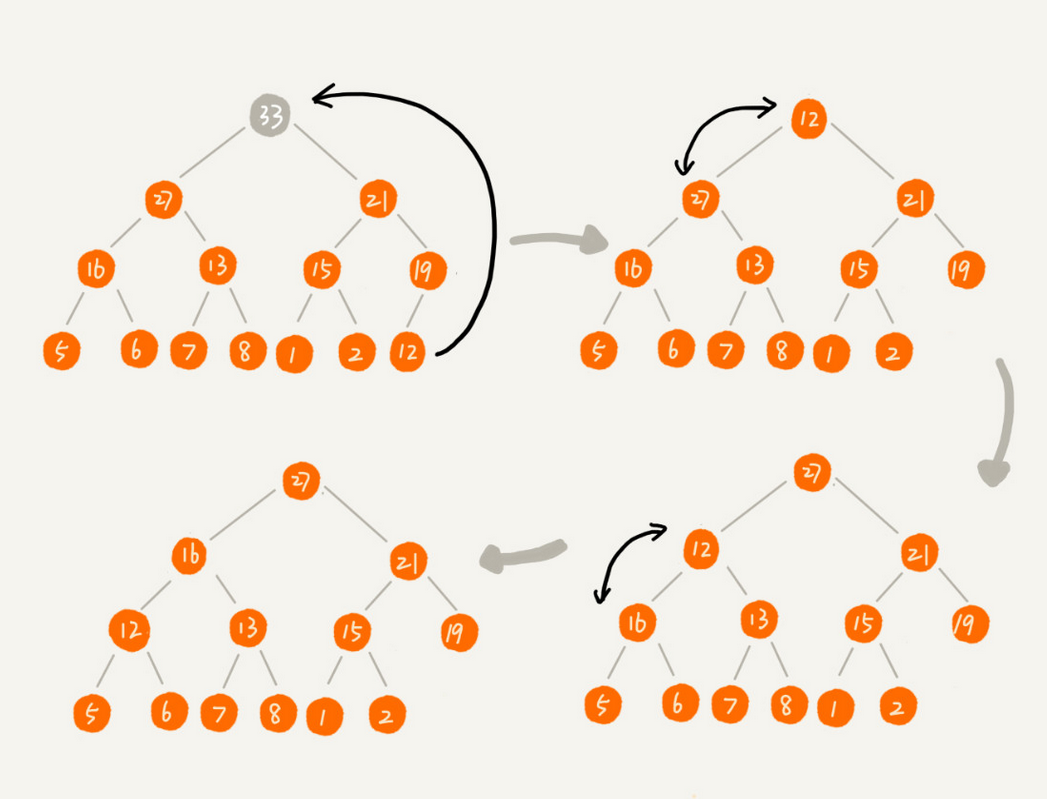• 选择排序：  每一趟从待排序的数据元素中选出最小（或最大）的一个元素，顺序放在已排好序的数列的最前（或者最后） 直到全部待排序的数据元素排完。 选择排序是不稳定的排序方法。 下面是用C语言实现的...
选择排序：

每一趟从待排序的数据元素中选出最小（或最大）的一个元素，顺序放在已排好序的数列的最前（或者最后）
直到全部待排序的数据元素排完。 选择排序是不稳定的排序方法。

下面是用C语言实现的选择排序方法将数据进行升序（正序）排序：

#include<stdio.h>

void main()
{
void selectionSort(int a[], int n); // 排序方法的声明

int a = {10, 3, 7, 1, 4, 6, 5, 2, 8, 9};

selectionSort(a, 10);

// 将排序后的结果输出

for(int i = 0; i < 10; i++)
{
printf("%d  ", a[i]);
}
}

void selectionSort(int a[], int n)
{
int i, j, k, t;

for(i = 0; i < n - 1; i++)
{
k = i;

for(j = i + 1; j < n; j++)
{
if(a[j] < a[k])
{
k = j;
}

}

t = a[k]; a[k] = a[i]; a[i] = t;

}
}



展开全文语言
• 选择排序（Selection Sort） 选择排序的基本思想：每次会从未排序区间中找到最小的元素，将其放到已排序区间的末尾。主要有简单选择排序，堆排序。...// 简单选择排序c实现，a表示数组，n表示数组大小 /**...
选择排序（Selection Sort）
选择排序的基本思想：每次会从未排序区间中找到最小的元素，将其放到已排序区间的末尾。主要有简单选择排序，堆排序。
简单选择排序
简单选择排序的基本思想：每次从未排序区间中找到一个最小的元素，与未排序区间的第一个元素交换，这样每次就可以确定一个元素的最终的位置，重复n-1，就完成了对n个数据的排序。
#include <stdio.h>
#include <stdlib.h>
// 简单选择排序c实现，a表示数组，n表示数组大小
/**
* Author: gamilian
*/
void selection_sort(int a[], int n){
for (int i=0; i < n - 1; i++){ 	//n-1次排序，n-1个元素确定了位置，最后一个元素自然确定了位置。
int min = i;				//最小值的位置
for (int j = i; j < n; j++)
if(a[j] < a[min]){		//打擂台形式找出未排序序列最小值下标
min = j;
}
if (min != i){				//若最小值下标改变则交换，用if减少了不必要的交换（最小值下标未变）
int temp = a[i];
a[i] = a [min];
a[min] = temp;
}
}
}


#	简单选择排序python实现
"""
Author: gamilian
"""
def selection_sort(a):
'''简单选择排序
args:
a: List[int]
'''
length = len(a)
if length <= 1:
return
for i in range(0, length - 1):
min = i
for j in range(i, length):
if a[j] < a[min]:
min = j
if min != i:
a[min], a[i] = a[i], a[min]

算法的稳定性：选择排序每次都要找剩余未排序元素中的最小值，并和前面的元素交换位置，这样破坏了稳定性。所以选择排序是一种不稳定的排序算法。
空间复杂度：从实现过程可以很明显地看出，简单选择排序算法的运行并不需要额外的存储空间，所以空间复杂度是 O(1)，也就是说，这是一个原地排序算法。
时间复杂度：简单选择排序的最好情况时间复杂度、最坏情况和平均情况时间复杂度都为 O(n^2)。
堆排序（Heap Sort）
堆
堆的性质：

堆是一个完全二叉树；

堆中每一个节点的值都必须大于等于（或小于等于）其子树中每个节点的值，即堆中每个节点的值都大于等于（或者小于等于）其左右子节点的值

对于每个节点的值都大于等于子树中每个节点值的堆，我们叫作“大顶堆”。对于每个节点的值都小于等于子树中每个节点值的堆，我们叫作“小顶堆”。

堆的表示：由于堆是一颗完全二叉树，而完全二叉树比较适合用数组来存储。用数组来存储完全二叉树是非常节省存储空间的。因为我们不需要存储左右子节点的指针，单纯地通过数组的下标，就可以找到一个节点的左右子节点和父节点。如图所示：从图中我们可以看到，数组中下标为 i 的节点的左子节点，就是下标为 i∗2 的节点，右子节点就是下标为 i∗2+1 的节点，父节点就是下标为 i/2 的节点，最后一个非叶节点编号为n/2。
堆化（heapify）：也就是在一个堆中插入一个元素到堆的最后，然后调整新的完全二叉树，让其重新满足堆的特性的过程。
从下往上堆化：我们可以让新插入的节点与其父节点对比大小。如果不满足子节点小于等于父节点的大小关系，我们就互换两个节点。一直重复这个过程，直到父子节点之间满足刚说的那种大小关系。#include <stdio.h>
#include <stdlib.h>
// 堆化c实现
/**
* Author: gamilian
*/
typedef int HeapDataType;
typedef struct Heap{
HeapDataType* data;
int count;  	//	堆中已经存储的数据个数
int MaxSize; 	//	堆可以存储的最大数据个数
}HP;

//对于low-high的元素向上调整，low一般为1，high表示欲调整节点的下标
void upAdjust(HP* hp, int low, int high){
int i = high, j = i / 2;				//i为欲调整节点的下标，j为其父节点
while (j >= low){						//父节点在[low, high]内
if (hp->data[j] < hp->data[i]){		//不满足子节点小于等于父节点的大小关系，则交换
int tmp = hp->data[i];
hp->data[i] = hp->data[j];
hp->data[j] = tmp;
i = j;							//保持原来状态
j = i / 2;
}
else
break;
}
}

//插入元素自下往上堆化
void heapify(HP* hp, HeapDataType value){
if (hp->count >= hp->MaxSize)
return; // 堆满了
hp->data[++hp->count] = value;
upAdjust(hp, 1, hp->count);
}


#	堆化python实现，见最后

删除堆顶元素：假设我们构造的是大顶堆，堆顶元素就是最大的元素。当我们删除堆顶元素之后，我们将我们把最后一个节点放到堆顶，然后利用同样的父子节点对比方法。对于不满足父子节点大小关系的，互换两个节点，并且重复进行这个过程，直到父子节点之间满足大小关系为止，即从上往下的堆化方法。//删除堆顶元素c实现
/**
* Author: gamilian
*/
//对于low-high的元素向下调整，low为欲调整节点的下标，high一般为堆中最后一个元素的下标
void downAdjust(HP* hp, int low, int high){
int i = low, j = i * 2;					//i为欲调整节点的下标，j为其左孩子
while (j <= high){						//孩子节点在[low, high]内
if (j + 1 <= high && hp->data[j] < hp->data[j + 1])
j = j + 1;						//右孩子存在且左孩子小于右孩子
if (hp->data[j] > hp->data[i]){		//不满足子节点小于等于父节点的大小关系，则交换
int tmp = hp->data[i];
hp->data[i] = hp->data[j];
hp->data[j] = tmp;
i = j;							//保持原来状态
j = i * 2;
}
else
break;
}
}

//删除堆顶元素
void deleteTop(HP* hp){
if (hp->count < 1)
return;		//堆为空
hp->data = hp->data[hp->count--];
downAdjust(hp , 1, hp->count);
}

#	删除堆顶元素python实现，见最后

堆排序（Heap Sort）
1、建堆
我们首先将数组原地建成一个堆。所谓“原地”就是，不借助另一个数组，就在原数组上操作。建堆的过程，有两种思路。

第一种是借助之前在堆中插入一个元素的思路。尽管数组中包含 n 个数据，但是我们可以假设，起初堆中只包含一个数据，就是下标为 1 的数据。然后，我们调用前面讲的插入操作，将下标从 2 到 n 的数据依次插入到堆中。这样我们就将包含 n 个数据的数组，组织成了堆。

//第一种方法初始化堆,heapify算法
/**
* Author: gamilian
*/
void initMaxHeap1(HP* hp, int size, HeapDataType* arr){
hp->MaxSize = size;
hp->data = (HeapDataType*)malloc((hp->MaxSize + 1) * sizeof(HeapDataType));//从1开始存储
hp->data = arr;
hp->count = 1;
//把arr数组的值一个个插入到这个堆。
for (int i = 1; i < size; i++)
{
heapify(hp , arr[i]);
}
}

#	第一种方法初始化堆，python实现，见最后


第二种实现思路，跟第一种截然相反，第一种建堆思路的处理过程是从前往后处理数组数据，并且每个数据插入堆中时，都是从下往上堆化，而第二种实现思路，是从后往前处理数组，并且每个数据都是从上往下堆化。

//第二种方法初始化堆
/**
* Author: gamilian
*/
void initMaxHeap2(HP* hp, int size, HeapDataType* arr){
hp->MaxSize = size;
hp->data = (HeapDataType*)malloc((hp->MaxSize + 1) * sizeof(HeapDataType));//从1开始存储
//把arr数组的值赋给这个堆
for (int i = 0; i < size; i++)
{
hp->data[i + 1] = arr[i];
}
hp->count = size;

//整合堆操作,向下调整
for (int i = hp->count / 2; i > 0; i--)
{
downAdjust(hp, i, hp->count);
}
}

#	第二种方法初始化堆，python实现，见最后

2、排序
建堆结束之后，数组中的数据已经是按照大顶堆的特性来组织的。数组中的第一个元素就是堆顶，也就是最大的元素。我们把它跟最后一个元素交换，那最大元素就放到了下标为 n 的位置。这个过程有点类似上面讲的“删除堆顶元素”的操作，当堆顶元素移除之后，我们把下标为 n 的元素放到堆顶，然后再通过堆化的方法，将剩下的 n−1 个元素重新构建成堆。堆化完成之后，我们再取堆顶的元素，放到下标是 n−1 的位置，一直重复这个过程，直到最后堆中只剩下标为 1 的一个元素，排序工作就完成了。
// 堆排序c实现,a表示数组，n表示数组大小
/**
* Author: gamilian
*/
void heap_sort(int a[], int n){
HP hp;
initMaxHeap1(&hp, n, a);		//建堆
for (int i = n; i > 1; i--){	//交换堆顶与最后一个元素，并不断调整
int tmp = hp.data;
hp.data = hp.data[i];
hp.data[i] = tmp;
downAdjust(&hp, 1, i-1);
}
for (int i = 0; i < n; ++i)
{
a[i] = hp.data[i + 1];
}
}

#	堆排序python实现
"""
Author: gamilian
"""
from typing import Optional, List

class Heap:
def __init__(self, capacity: int):
self._data =  * (capacity + 1)
self._capacity = capacity
self._count = 0

@classmethod
def _parent(cls, child_index: int) -> int:
"""父节点索引"""
return child_index // 2

@classmethod
def _left(cls, parent_index: int) -> int:
"""左孩子索引"""
return 2 * parent_index

@classmethod
def _right(cls, parent_index: int) -> int:
"""右孩子索引"""
return 2 * parent_index + 1

#	向上调整
def _upAdjust(self) -> None:
i, parent = self._count, Heap._parent(self._count)
while parent and self._data[i] > self._data[parent]:
self._data[i], self._data[parent] = self._data[parent], self._data[i]
i, parent = parent, Heap._parent(parent)

#	向下调整
@classmethod
def _downAdjust(cls, a: List[int], count: int, root_index: int = 1) -> None:
i = larger_child_index = root_index
while True:
left, right = cls._left(i), cls._right(i)
if left <= count and a[i] < a[left]:
larger_child_index = left
if right <= count and a[larger_child_index] < a[right]:
larger_child_index = right
if larger_child_index == i: break
a[i], a[larger_child_index] = a[larger_child_index], a[i]
i = larger_child_index

# 堆化
def heapify(self, value: int) -> None:
if self._count >= self._capacity: return
self._count += 1
self._data[self._count] = value
self._upAdjust()

#	删除堆顶元素
def deleteTop(self) -> Optional[int]:
if self._count:
result = self._data
self._data = self._data[self._count]
self._count -= 1
Heap._downAdjust(self._data, self._count)
return result

# 第一种方法建堆
@classmethod
def build_heap_1(cls, a: List[int]) -> None:
"""数组索引需要从1开始"""
heap =cls(len(a) - 1)
heap._data = None
heap._data = a
heap._count = 1
for i in range(2, len(a)):
heap.heapify(a[i])
for i in range(len(a)):
a[i] = heap._data[i]

#第二种方法建堆
@classmethod
def build_heap_2(cls, a: List[int]) -> None:
"""数组索引需要从1开始"""
for i in range((len(a) - 1) // 2, 0, -1):
cls._downAdjust(a, len(a) - 1, i)

@classmethod
def sort(cls, a: List[int]) -> None:
"""数组索引需要从1开始"""
cls.build_heap_1(a)
k = len(a) - 1
while k > 1:
a, a[k] = a[k], a
k -= 1
cls._downAdjust(a, k)

def __repr__(self):
return self._data[1: self._count + 1].__repr__()

def heap_sort(a):
a.insert(0, None)
Heap.sort(a)
a[:] = a[1:]

算法的稳定性：堆排序每次筛选时，可能把后面相同关键字的元素调整到前面，这样破坏了稳定性。所以选择排序是一种不稳定的排序算法。
空间复杂度：从实现过程可以很明显地看出，堆排序算法的运行并不需要额外的存储空间，所以空间复杂度是 O(1)，也就是说，这是一个原地排序算法。
注：第一种方法建堆，需要额外的存储空间，空间复杂度O(n)
时间复杂度：堆排序的最好情况时间复杂度、最坏情况和平均情况时间复杂度都为 O(nlogn)。


展开全文• 选择排序的原理： 第一次从待排序的数据元素中选出最小（或最大）的一个元素，存放在序列的起始位置，然后再从剩余的未排序元素中寻找到最小（大）元素，然后放到已排序的序列的末尾。以此类推，直到全部待排序的...
选择排序的原理：

第一次从待排序的数据元素中选出最小（或最大）的一个元素，存放在序列的起始位置，然后再从剩余的未排序元素中寻找到最小（大）元素，然后放到已排序的序列的末尾。以此类推，直到全部待排序的数据元素的个数为零。选择排序是不稳定的排序方法。

时间复杂度：O(n^2）

代码实现如下：

#include <stdio.h>

void swap(int *a, int *b)
{
int tmp;
tmp = *a;
*a = *b;
*b = tmp;
}

void selectSort(int arr[], int n)
{
int i, j;

for (i = 0;i < n; i++) {
int minIndex = i;
for (j = i; j < n; j++) {
if (arr[j] < arr[minIndex])
minIndex = j;
}
swap(&arr[i], &arr[minIndex]);
}
}

int main(void)
{
int arr = {3, 2, 5, 4, 1};
selectSort(arr, 5);
int i;
for (i = 0; i < 5; i++)
printf("%d  ", arr[i]);
printf("\n");
return 0;
}


展开全文• 简单选择排序思路是从i开始遍历，选择最小值插入到arr[ i ], 下一次从i + 1处遍历，直到遍历完成。思路和冒泡排序刚好相反，冒泡排序是每次遍历时选取最大值，简单选择遍历每次选取最小值，冒泡排序过程的确像水中...
简单选择排序思路是从i开始遍历，选择最小值插入到arr[ i ], 下一次从i + 1处遍历，直到遍历完成。思路和冒泡排序刚好相反，冒泡排序是每次遍历时选取最大值，简单选择遍历每次选取最小值，冒泡排序过程的确像水中冒泡的样子，但要是这样，简单选择排序不是应该叫做沉底排序吗？每次遍历，最小值沉了下去。

代码比较简单，遍历选取最小值。下面是c语言实现：

//两数交换
void swap(int *a, int *b)
{
*a = *a ^ *b;
*b = *a ^ *b;
*a = *a ^ *b;
}

//简单选择排序
void selectSort(int *arr, int numsSize)
{
int i, j, minLoc, minValue;
minValue = arr;

for (i = 0; i < numsSize - 1; i++)
{
//保存当前最小值及所在位置
minLoc = i;
minValue = arr[i];

//查找最小值
for (j = i + 1; j < numsSize; j++)
{
if (minValue > arr[j])
{
minValue = arr[j];
minLoc = j;
}
}

//保存
if (i != minLoc)
swap (arr + i, arr + minLoc);
}
}

=============================================================================================

Linux应用程序、内核、驱动、后台开发交流讨论群(745510310)，感兴趣的同学可以加群讨论、交流、资料查找等，前进的道路上，你不是一个人奥^_^。


展开全文• 选择排序算法时间复杂度O（n*n），在内嵌生成十万随机数生成下，选择算法在该条件下平均运行时间在12s多一点。 #include #include #define LEN 100000 //数据量：十万 void selectSort(int arr[],int len); ...
• 选择排序的基本思想是：每一趟从待排序的记录中选出关键字最小的记录，按顺序放在已排序的记录序列的最后，直到全部排完为止。 括号内为已排完序的，每一趟结束待排序记录的个数就少一个。 算法属性： 时间复杂度为...数据结构 算法
• 树形排序即简单选择排序的改进，第一躺比较后对记录大小进行记录，以后不再逐一比较，堆排序 (Heap Sort) 是一种树形选择排序。 堆实质上是满足如下性质的完全二叉树：树中所有非终端结点的值均不大于（或不小于） ...数据结构
• ******由小到大排序****** 算法思想: ①从左往右遍历待排序列找到其中最小的元素，然后和待排序列的第一个元素交换位置；②把从第二个元素开始的剩余元素看作新的待排序列，重复以上操作，直至最后一个元素。 下面...
• 选择排序(Selection sort)是一种简单直观的排序算法。它的工作原理如下。首先在未排序序列中找到最小（大）元素，存放到排序序列的起始位置，然后，再从剩余未排序元素中继续寻找最小（大）元素，然后放到已排序序列...lua
• 思路：首先，找到数组中最小的那个元素，其次，将它和数组的第一个...不断的选择剩余元素中的最小者，所以称为选择排序C/C++实现 void SelectionSort(int a[], int size) { for (int i = 0; i < size - 1;...C#实现
• // #include<stdio.h> #include<windows.h> #define SWAMP(a,b) (a)+=(b),(b)=(a)-(b),(a)-=(b) void SwampTwoInt(int *a,int *b) { int temp=*a; *a=*b; *b=temp;...void Choos...
• #include int main(int argc, const char * argv[]) { ... //选择排序  int infos={9,3,1,6,8};  int temp;  for (int i=0; i4; i++) { //0————n-2,i  for (int j=i+1; j5; j
• ## 直接选择排序C代码

千次阅读 2009-09-22 16:02:00
void StraightSelectionSort(int array[], unsigned int n){ /* 注：关键字值类型为int，数组的索引是从... 第1趟排序从array【0， n - 1】中找到下标为k的关键字最小值，把array【k】和 array【0】交换。现在无序区为a算法
• #include&amp;lt;stdio.h&amp;gt; #include&amp;lt;stdlib.h&amp;gt; #define N 10 void SelectionSort(int* a,int n,int i) { if(i&amp;gt;=n-1) { return; } else{ ... ...算法设计 C语言...